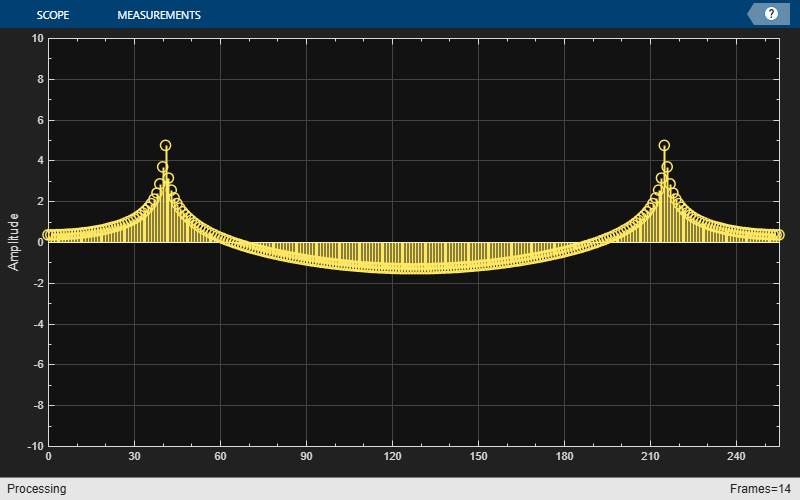write

Write data to buffer

Description

example

nOverrun = write(asyncBuff,x) writes the input array, x, to the buffering object, asyncBuff, and returns the number of samples overrun, nOverrun.

Examples

collapse all

Write a sine wave of variable frame size to the buffer. Compute the FFT of the sine wave and visualize the result on an array plot.

Initialize the dsp.AsyncBuffer, dsp.ArrayPlot, and dsp.FFT System objects.

asynBuff = dsp.AsyncBuffer;
plotter = dsp.ArrayPlot;
fftObj = dsp.FFT('FFTLengthSource','Property','FFTLength',256);

The sine wave is generated using the sin function in MATLAB. The start and finish variables mark the start and finish indices of each frame. If enough data is cached, read from the buffer and perform the FFT. View the FFT on an array plot.

start = 1;

for Iter = 1 : 2000
numToWrite = randi([200,800]);
finish = start + numToWrite;

inputData = sin(start:finish)';
start = finish + 1;

write(asynBuff,inputData);
X = abs(fftObj(x));
plotter(log(X));
end
endInput Arguments

collapse all

Async buffer, specified as a dsp.AsyncBuffer System object.

Data written to the buffer, specified as a vector or a matrix. The maximum number of rows in the buffer is determined by the Capacity property of asyncBuff. The number of channels in the buffer is determined by the second dimension of the first data written to the buffer. Successive data inputs can vary in the number of rows, but the number of channels must remain fixed. To change the number of channels, you must call release on the buffer.

For example, the following is accepted:

asyncBuff = dsp.AsyncBuffer;
% First call to write
write(asyncBuff,randn(15,5));
% Add more data with a different number of rows
write(asyncBuff,randn(25,5));
write(asyncBuff,randn(5,5));

The following is not accepted and errors out:

asyncBuff = dsp.AsyncBuffer;
% First call to write
write(asyncBuff,randn(15,5));
% Add more data with a different number of columns
write(asyncBuff,randn(15,15));

To change the number of channels, call release on the buffer.

asyncBuff = dsp.AsyncBuffer;
% First call to write
write(asyncBuff,randn(15,5));
release(asyncBuff)
% Add more data with a different number of columns
write(asyncBuff,randn(15,15));

Data Types: single | double | int8 | int16 | int32 | int64 | uint8 | uint16 | uint32 | uint64 | logical
Complex Number Support: Yes

Output Arguments

collapse all

Number of samples overrun in the current call to write. The number of samples overrun is the number of unread samples overwritten. If x is a multichannel input, then nOverrun is the number of rows of data overrun.

Data Types: int32

Objects

Introduced in R2017a

DSP System Toolbox DocumentationGet trial now